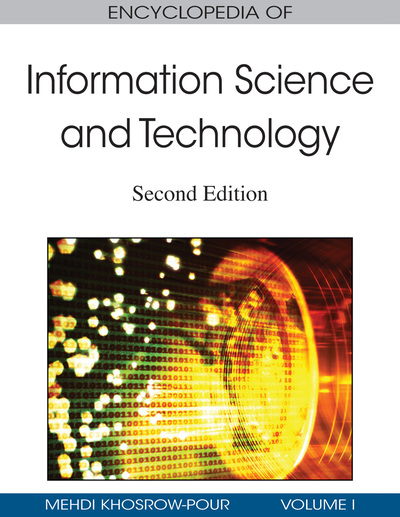# Attribute Grammars and Their Applications

Krishnaprasad Thirunarayan (Wright State University, USA)
DOI: 10.4018/978-1-60566-026-4.ch046
Available
\$37.50
No Current Special Offers

## Abstract

Attribute grammars are a framework for defining semantics of programming languages in a syntax-directed fashion. In this chapter, we define attribute grammars, and then illustrate their use for language definition, compiler generation, definite clause grammars, design and specification of algorithms, and so forth. Our goal is to emphasize its role as a tool for design, formal specification and implementation of practical systems, so our presentation is example rich.
Chapter Preview
Top

## Introduction

• Attribute grammars are a framework for defining semantics of programming languages in a syntax-directed fashion. In this chapter, we define attribute grammars, and then illustrate their use for language definition, compiler generation, definite clause grammars, design and specification of algorithms, and so forth. Our goal is to emphasize its role as a tool for design, formal specification and implementation of practical systems, so our presentation is example rich.

Top

## Background

The lexical structure and syntax of a language is normally defined using regular expressions and context-free grammars respectively (Aho, Lam, Sethi & Ullman et al., 2007,). Knuth (1968) introduced attribute grammars to specify static and dynamic semantics of a programming language in a syntax-directed way.

Let G = (N, T, P, S) be a context-free grammar for a language L (Aho et al., 2007). N is the set of non-terminals. T is the set of terminals. P is the set of productions. Each production is of the form A::= α, where A ∈ N and α ∈ (N U T)*. S ∈ N is the start symbol. An attribute grammar AG is a triple (G, A, AR), where G is a context-free grammar for the language, A associates each grammar symbol X ∈ N U T with a set of attributes, and AR associates each production R ∈ P with a set of attribute computation rules (Paakki, 1995). A(X), where X ∈ (N U T), can be further partitioned into two sets: synthesized attributes S(X) and inherited attributes I(X). AR(R), where R ∈ P, contains rules for computing inherited and synthesized attributes associated with the symbols in the production R.

Consider the following attribute grammar that maps bit strings to numbers. CFG = ({N},{0,1},P,N), where P is the left column of productions shown below. The number semantics is formalized by associating a synthesized attribute val with N, and providing rules for computing the value of the attribute val associated with the left-hand side N (denoted Nl) in terms of the value of the attribute val associated with the right-hand side N (denoted Nr), and the terminal.

```N::= 0 N.val = 0
N::= 1 N.val = 1
N::= N0 Nl.val = 2 * Nr.val
N::= N1 Nl.val = 2 * Nr.val + 1
```

An attribute grammar involving only synthesized attributes is called an S-attributed grammar. It is straightforward to parse a binary string using this grammar and then compute the value of the string using a simple top-down left-to-right traversal of the abstract syntax tree.

The above attribute grammar is not unique. One can construct a different S-attributed grammar for the same language and the same semantics as follows.

```N::= 0 N.val = 0, N.len = 1
N::= 1 N.val = 1, N.len = 1
N::= 0N Nl.val = Nr.val;
Nl.len = Nr.len +1
N::= 1N Nl.val = 2^ Nr.len + Nr.val;
Nl.len = Nr.len +1
```

Attribute grammars can be devised to specify different semantics associated with the same language. For instance, the bit string can be interpreted as a fraction by associating an inherited attribute pow to capture the left context—the length of the bit string between left of a non-terminal and the binary point, to determine the local value or the weight of the bit.

```F::= . N F.val = N.val, N.pow = 1
N::= 0 N.val = 0
N::= 1 N.val = (1 / 2^ N.pow)
N::= 0N Nl.val = Nr.val;
Nr.pow = Nl.pow +1
N::= 1N Nl.val = (1 / 2^ N.pow) + Nr.val;
Nr.pow = Nl.pow +1
```

## Key Terms in this Chapter

XML/XSLT: Extensible markup l,anguage is a meta-language for creating markup languages. XML is a subset of SGML. XHTML is an instance of XML. Extensible stylesheet language transformations is a language for manipulating XML documents.

Attribute Computation Rules: Rules used to compute attributes, usually defined in terms of other attributes and standard primitives.

Synthesized Attributes: These attributes pass information from leaves to the root of a parse tree.

DCG: A definite clause grammar is a Prolog built-in mechanism for implementing attribute grammars efficiently using difference lists.

Attribute Grammar: An attribute grammar is an extension of context-free grammar that enables definition of context-sensitive aspects of a language and its translation.

Machine-Processable Semantics: Metadata added to the documents to enable machines to understand and reason with text or multi-media content.

EBNF: Extended Backus Naur formalism is an extension of context-free grammar with regular expression operations for defining context-free languages. It provides a more concise syntax specification.

Inherited Attributes: These attributes pass information from root to the leaves of a parse tree, or sideways among siblings.

## Complete Chapter List

Search this Book:
Reset